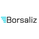TradingView
1484 views
Plot of equilibrium price of Relative Strength .
Instead of the RS ratio, this is the plot of the price that will make the RS ratio equal to zero, we call this the equilibrium price.
About the equilibrium price plot:
- When the plot is below the symbol price plot, it means stock is performing above the benchmark
- When the plot is above the symbol price plot, it means stock is underperforming in comparison to the benchmark
- When the plot is close (or equal) to the symbol price plot, it means that RS is near the zero line, either crossing over or crossing below

The latest equilibrium is displayed over the plot. This gives a visual and quantitative idea of how far above or below the equilibrium price is in comparison to the current price.

In the above example, we see that HDFC Bank which was performing above the benchmark (trading above equilibrium price) whipsawed a lot (price near equilibrium price), before swaying down during the COVID-19 fall in the market (price is trading below the equilibrium price) . Currently, the equilibrium price is 1136, but HDFC Bank is trading below this price at 1033, around 100 points below.
Release Notes:
• Equilibrium Price, upto 2 places of decimal
• Minor typo fix
Open-source script

In true TradingView spirit, the author of this script has published it open-source, so traders can understand and verify it. Cheers to the author! You may use it for free, but reuse of this code in a publication is governed by House Rules. You can favorite it to use it on a chart.

Want to use this script on a chart?

## CommentsHi, good idea, thank you. I see that the labeled prices rounds up. How can we remove rounding up to see the correct two digit price? Such as 5,35.
ReplybharatTrader
@bharatTrader, like presently it is calculated from 123 days right, so if you have one more to calculate from adaptive such as 149 today ,we can see two lines on price charts at a time with the price label, it will be awesome for entry and exit (like if someone has position way above zero line,till crossing zero down ,what will be probable price he will know decide to remain in trade or exit...👍
Reply
Profile Profile Settings Account and Billing Referred friends Coins My Support Tickets Help Center Private Messages Chat Sign Out